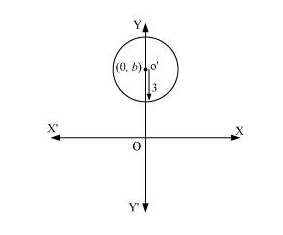# Form the differential equation of the family of circles having centre on y-axis and radius 3 units.

Question:

Form the differential equation of the family of circles having centre on y-axis and radius 3 units.

Solution:

Let the centre of the circle on y-axis be (0, b).

The differential equation of the family of circles with centre at (0, b) and radius 3 is as follows:

$x^{2}+(y-b)^{2}=3^{2}$

$\Rightarrow x^{2}+(y-b)^{2}=9$                      ...(1)Differentiating equation (1) with respect to x, we get:

$2 x+2(y-b) \cdot y^{\prime}=0$

$\Rightarrow(y-b) \cdot y^{\prime}=-x$

$\Rightarrow y-b=\frac{-x}{y^{\prime}}$

Substituting the value of (y – b) in equation (1), we get:

$x^{2}+\left(\frac{-x}{y^{\prime}}\right)^{2}=9$

$\Rightarrow x^{2}\left[1+\frac{1}{\left(y^{\prime}\right)^{2}}\right]=9$

$\Rightarrow x^{2}\left(\left(y^{\prime}\right)^{2}+1\right)=9\left(y^{\prime}\right)^{2}$

$\Rightarrow\left(x^{2}-9\right)\left(y^{\prime}\right)^{2}+x^{2}=0$

This is the required differential equation.